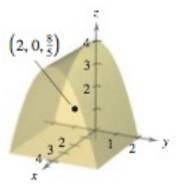Chapter 14.6, Problem 46E

Chapter
Section
Textbook Problem

Think About It The center of mass of a solid of constant density is shown in the figure. In Exercises 43-46, make a conjecture about how the center of mass ( x ¯ , y ¯ , z ¯ ) will change for the nonconstant density ρ ( x , y , z ) . Explain. (Make your conjecture without performing any calculations.)ρ ( x , y , z ) = k x z 2 ( y + 2 ) 2

To determine
A explanation about how the center of mass (x¯,y¯,z¯) will change for the non-constant density ρ(x,y,z)=kxz2(y+2)2 by the center of mass (2,0,85) of a solid of constant density.

Explanation

Given:

The center of mass of a solid of constant density is shown in figure:

Explanation:

The given center of mass of solid of constant density is (2,0,85).

So, x=2, y=0, and z=85

Still sussing out bartleby?

Check out a sample textbook solution.

See a sample solution

The Solution to Your Study Problems

Bartleby provides explanations to thousands of textbook problems written by our experts, many with advanced degrees!

Get Started

Find more solutions based on key concepts